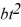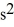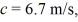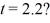# [Solved] The Position of an Object as a Function of Time

Question 29
Multiple Choice

## The position of an object as a function of time is given by x =- ct, where b = 2.0 m/andand x and t are in SI units. What is the instantaneous velocity of the object whenA) 1.7 m/s
B) 2.1 m/s
C) 2.3 m/s
D) 2.7 m/s

10+ million students use Quizplus to study and prepare for their homework, quizzes and exams through 20m+ questions in 300k quizzes.

### Physics & Astronomy

Explore our library and get Introduction to Physical Science Homework Help with various study sets and a huge amount of quizzes and questions

121

Study sets

3.1K

Quizzes

233.6K

Questions

Upload material to get free accessInvite a friend and get free accessSubscribe and get an instant access